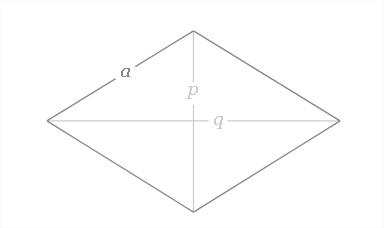# C Program To Calculate Perimeter Of Rhombus | C Programs

C Program to calculate the perimeter of a rhombus – In this particular article, we will discuss the several ways to calculate the perimeter of a rhombus in C programming.

The methods used to do so are as follows:

• Using Standard Method
• Using Functions
• Using Pointers
• Using Macros

As we all know, a rhombus is a 2-dimensional quadrilateral figure whose sides are all equal in nature. The opposite sides of a rhombus are parallel as well.

A rhombus would look like this:As you can see, p and q are the two diagonals of the rhombus. Since the diagonals of a rhombus cut each other at right angles, it’s easy to evaluate the side of a rhombus.

Applying the Pythagoras Theorem,

a = sqrt(p^2 + q^2)

Thus, the perimeter of a rhombus = 4a.

C Program to calculate the perimeter of the rhombus in three different ways. Check out our other C Beginner programs. We have written the code in three different ways, with sample outputs and example programs.

The multitude of methods with which the perimeter of a rhombus can be calculated in C Programming is as follows:

## Using Standard Method

1)The value of the entered side of rhombus will store into variable”side”

2)Using perimeter=4*side formula, we calculate the perimeter value

output:

### Using Functions

1)p=perimeter(s) using this code we are calling the perimeter function.

2)float perimeter(float s) returns the perimeter value and perimeter value will be assigned to p.

output:

#### Using Pointers

1)We are passing the addresses of s,p variables using perimerer(&s,&p) function.

2)void perimeter(float *s, float *p) retrieve  the values at  that addresses and calculate the perimeter value

3)The perimeter value will be stored into pointer variable *p

output:

##### Using Macros

1)Here 4*s formula was defined with the name area(s)

2)area(s) replaced with that expression given at #define.

output: